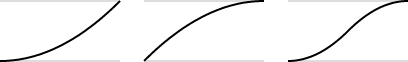# Supported Tweener Easing Types

The easiest way to visualize the concept of Scripted Entity Tweener easing types is to see it on a graph that depicts a change in position over time. The resulting line can be thought of as speed, though it isn’t necessarily so.

The movement of objects in the natural world is rarely uniform. A rocket ship blasting off from the ground accelerates slowly as it first gains momentum, and then rapidly accelerates as it shoots toward the sky. If you viewed this motion on a position and time graph, it might look like a quartic or quintic easing in function. A bouncy ball dropped from a height accelerates toward the ground and hits it at a certain velocity. The ball bounces back up at that velocity and decelerates as it nears the top of its arc, and gravity pulls it back down for another bounce. Viewed on a position and time graph, looks like the bounce easing out function.

O3DE supports the following tweener ease methods as easing in, easing out, and easing in-out functions (ease type).

Note:
The following images are referenced from http://easings.net.

Linear Does not ease in or out. No acceleration or deceleration. Constant movement, such as a conveyor belt in motion.Quadratic Easing in function starts at a zero velocity, then accelerates. Easing out function begins at a certain velocity and decelerates to zero. Easing in-out function starts at zero velocity, accelerates until the halfway point, and then decelerates to zero. The following graphs show quadratic ease in, quadratic ease out, and quadratic ease in-out functions.Cubic Similar to quadratic, but with a steeper curve, which indicates a slower rate of acceleration or deceleration at first, followed by a rapid acceleration or deceleration. The following graphs show cubic ease in, cubic ease out, and cubic ease in-out functions.Quart Similar to cubic, but with an even slower rate of acceleration or deceleration followed by an even faster rate of acceleration or deceleration. The following graphs show quartic ease in, quartic ease out, and quartic ease in-out functions.Quint Similar to quart, but with an even slower rate of acceleration or deceleration followed by an even faster rate of acceleration or deceleration. The following graphs show quintic ease in, quintic ease out, and quintic ease in-out functions.Sine Based on a sine or cosine function. Gentle easing in or easing out, with an almost constant speed like the linear function. The following graphs show sinusoidal ease in, sinusoidal ease out, and sinusoidal ease in-out functions.Exponential Similar to cubic. The following graphs show exponential ease in, exponential ease out, and exponential ease in-out functions.Circular Based on the equation for half of a circle. The graph in the following image is stretched vertically so that you can see how the graph looks like a part of a circle. The following graphs show circular ease in, circular ease out, and circular ease in-out functions.Elastic An example of an elastic easting out function is a plucked guitar string. The string moves up and down with decreasing frequency until it comes to a rest. Easing in is the same motion but in reverse. The following graphs show elastic ease in, elastic ease out, and elastic ease in-out functions.Back An example of a back easing in function is a toy car pulled backward to wind the springs, and then released. The following graphs show back ease in, back ease out, and back ease in-out functions.Bounce Depicts a bouncing motion. For example, a rubber ball dropped from a height displays a bounce ease out function as it bounces and eventually comes to rest. The following graphs show bounce ease in, bounce ease out, and bounce ease in out functions.